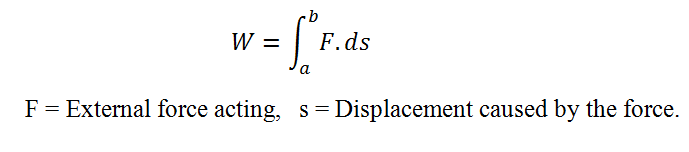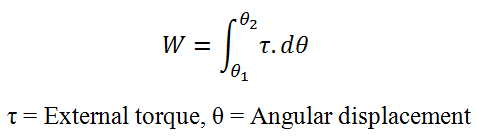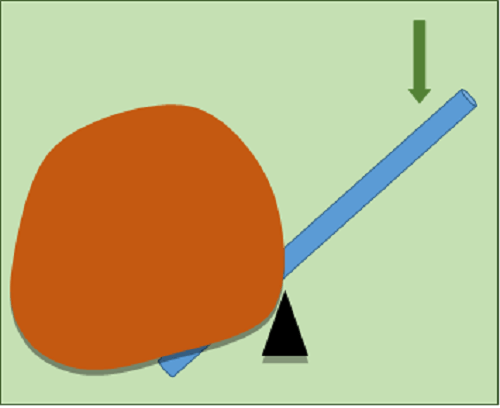# Difference Between Work and Power

## Main Difference – Work vs Power

Work and Power are two closely related terms discussed in physics. But these terms cannot be used interchangeably. For an example, when we express the capacity of a power plant or windmill we express it in either kW or MW or GW. There is no point in expressing the capacity of a power plant in either kWh or MWh or GWh. However, when we want to express our domestic energy consumption, we express it in kWh. The main difference between work and power is that work is the amount of energy transferred when an object is moved by an external force whereas power is the rate of work done.

## What is Work

Work is the amount of energy transferred when an object is moved by an unbalanced external force acting on it. It is a scalar quantity. Its SI unit is the Joule (J).

Work is done in several ways.

Ex: when an object is rotated by an external torque, when a gas is compressed or expanded, when a body is moved by an external force acting on it.

The work done by an external force acting on a body can be defined as the integral of the scalar product of the force and the displacement caused by that force. This work done (W) can be computed using the equation,The work done by an external torque acting on a body can be defined as the integral of the scalar product of the torque and the angular displacement caused by that torque. This work done (W) can be expressed as,The work done (W), when a gas is compressed at a constant pressure is given by, W= P (ΔV)

Where, P- Pressure, ΔP-Change in the volume.

When we deal with small energy values, eV is used as the unit. It is equal to the work done, on an electron when it is accelerated through a potential difference of 1V.

1eV= 1.602 J

When we deal with large quantities, kWh, MWh, GWh or TWh are used.## What is Power

Power is a very important concept in physics. It is the rate of work done. In other words, power is the amount of work done per unit time. It is a scalar quantity. The SI unit of measuring power is the Joule per second (Js-1) or the Watt (W). The concept of power is important when the working capacity of a water heater, electric oven, light bulb, fan, or a generator is expressed. This is because there is no point in expressing the working capacity of such an equipment in terms of energy as the energy consumptions of them depend on the time. Energy consumption increases with time, but the power remains unchanged (approximately) over time as an equipment is designed to work at a predetermined power. So, one can easily calculate the energy consumption of an equipment if he knows its power. In addition, the capacity of a power plant or windmill is expressed in terms of power (in MW).

If the “work done” and “time taken” are known, the power could be computed using the following equation.

Power = Work Done/Time Taken

The power could be electrical or mechanical. The electrical power could be calculated using following equations.If the force acting on an object and the velocity of the object are known, the mechanical power can be calculated using the equation below.

P= F.V

Where,

F is the force acting on the object.

V is the velocity of the object.

The following equation can be used to calculate the mechanical power of a rotating body.

P= τ.ω

Where,

τ is the torque acting on the object.

ω is the angular velocity of the object.

The following list is important when we deal with power.

1W= 1J

1kW= 1000 W

1MW= 1000 kW

1GW= 1000 MW

1TW= 1000 GW## Difference Between Work and Power

### Definition of Work and Power:

Work: Work is the amount of energy transfer when an object is moved by an external force.

Power: Power is the rate of work done.

### Nature of the quantity:

Work: Work is a scalar quantity.

Power: Power is also a scalar quantity.

### SI Unit:

Work: Work is measured by Joule (J).

Power: Power is measured by Watt (W).

### In SI Base Units:

Work: SI Base unit of work is kgm2 s-2

Power: SI Base unit of power is kgm2s-3

### Other Units:

Work: Work is also measured in eV, kWh, MWh, GWh.

Power:Power is also measured in kW, MW, GW.Image Courtesy:

“Horse Power” by Own work, original version in German by Sgbeer – (CC BY-SA 3.0) via Commons Wikimedia